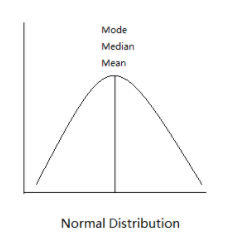## Mean

Mean, typically denoted by$\bar{x}$, measures the central value of the data set. It is the sum of all the values divided by the number of values.$\bar{x}=\frac{\sum_{i=1}^{n}{x_{i}}}{n}$Example

For example, let’s say in a class of 4 children, Alex, Beth, Charlie and Dawn, scored 3, 8, 21 and 9 for a test. As a teacher, you want to find out the average score for the class. We can use the mean formula to find this. In this case, for the sample$X=\{3,8,21,9\}$, the sample mean is$\bar{x}=\frac{\sum_{i=1}^{4}{x_{i}}}{4}=\frac{3+8+21+9}{4}=10.25$

Code

In code, this can be done as follows.

				
// create an array of doubles for our dataset
val values = doubleArrayOf(3.0, 8.0, 21.0, 9.0)

// get the array size
val n = values.size

var total = 0.0
var sample_mean : Double

// find the sum of all the values
for (i in 0..n-1) {
total += values.get(i)
}

sample_mean = total / n

println("Sample size: " + n)
println("Sample mean: " + sample_mean)


				
Sample size: 4
Sample mean: 10.25



In NM Dev, we can simply compute the sample of a dataset using the class Mean without having to come up with the algorithm ourselves.

				
// create an array of doubles for our dataset
val values = doubleArrayOf(3.0, 8.0, 21.0, 9.0)

// create the Mean object using the array that we created
val mean = Mean(values)

println("Sample size: " + mean.N())
println("Sample mean: " + mean.value())



				
Sample size: 4
Sample mean: 10.25



## Weighted Mean

Weighted mean allows certain values in the dataset to weigh more than others. Each value in the dataset can have its own weight$w_{i}$. It is the sum of all the values multiplied by each of their weights divided by the sum of all their weights.$\bar{x}^{*}=\frac{\sum_{i=1}^{n}{x_{i}w_{i}}}{\sum_{i=1}^{n}{w_{i}}}$

Example

Let’s say for example, a student took 5 subjects, English, Physics, Math, Economics and Computing and each subject has a different weight when calculating the student’s overall grade. The weights are as follows.

 Subject Weight English 2 Physics 3 Math 4 Economics 1 Computing 5

The student’s grades for each subject are as follows.

 Subject Grade English 88 Physics 94 Math 69 Economics 66 Computing 80

In order to find the student’s overall grade through the weighted mean formula, we must first multiply the grade for each subject with their respective weights.

 Subject Weighted Grade English 2 * 88 = 176 Physics 3 * 94 = 282 Math 4 * 69 = 276 Economics 1 * 66 = 66 Computing 5 * 80 = 400

We can then find the average of the weighted grades by finding the sum of all the weighted grades and then dividing it by the total weight.

Sum of weighted grades$=176+282+276+66+400=1200$

Total weight$=2+3+4+1+5=15$

Weighted mean$=1200/15=80$

Hence, the student’s overall grade is 80.

The above example can be defined mathematically as follows. The student’s grades for each subject are$X=\{88,94,69,66,80\}$ and each subject weighs differently in calculating the overall grade,$W=\{2,3,4,1,5\}$, the weighted sample mean is$\bar{x}^{*}=\frac{\sum_{i=1}^{5}{x_{i}w_{i}}}{\sum_{i=1}^{5}{w_{i}}}=\frac{88\times{2}+98\times{3}+69\times{4}+66\times{1}+80\times{5}}{2+3+4+1+5}=80$

Code

Translating this into code, we can use NM Dev’s class WeightedMean.

				
// create arrays for our dataset and our weights
val values = doubleArrayOf(88.0, 94.0, 69.0, 66.0, 80.0)
val weights = doubleArrayOf(2.0, 3.0, 4.0, 1.0, 5.0)

// create the WeightedMean object
val weighted_mean = WeightedMean(values, weights)

println("Sample weighted mean: " + weighted_mean.value())



				
Sample weighted mean: 80.0



## Thinking Time

Mean is used everywhere in the real world. Some use cases are:

• Finding the average salary in a certain field
• Finding the average expenditures of households
• Finding the average time it takes to run a race
• Finding the amount of hours spent on phones each week